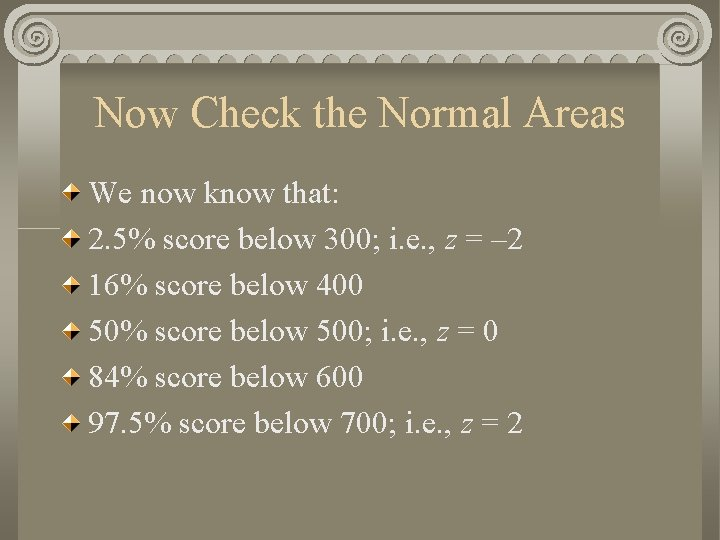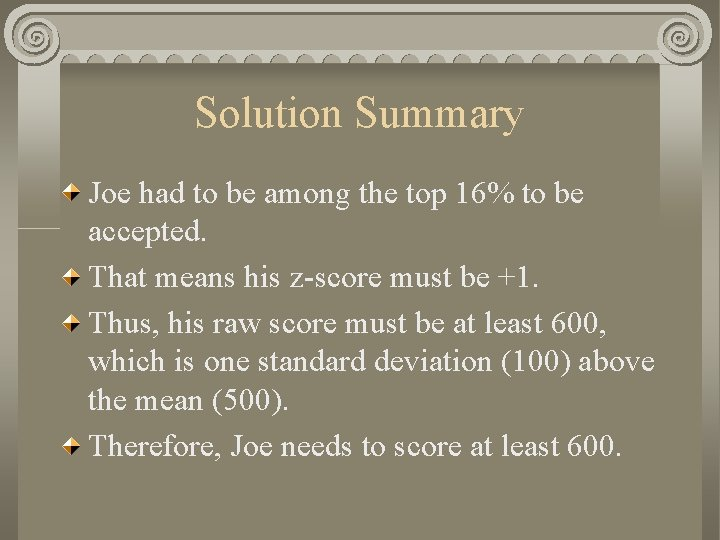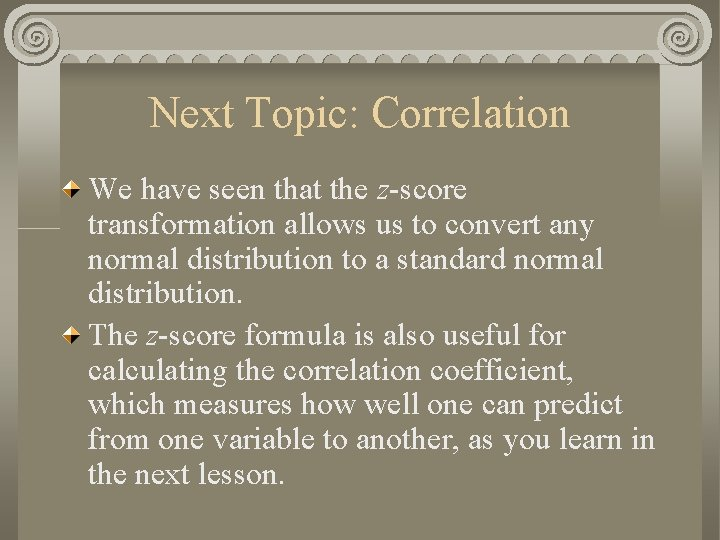Standard Normal Distribution The Classic BellShaped curve is

• Slides: 10Standard Normal Distribution The Classic Bell-Shaped curve is symmetric, with mean = median = mode = midpointStandard Normal DistributionProbabilities in the Normal Distribution The distribution is symmetric, with a mean of zero and standard deviation of 1. The probability of a score between 0 and 1 is the same as the probability of a score between 0 and – 1: both are. 34. Thus, in the Normal Distribution, the probability of a score falling within one standard deviation of the mean is. 68.More Probabilities The area under the Normal Curve from 1 to 2 is the same as the area from – 1 to – 2: . 135. The area from 2 to infinity is. 025, as is the area from – 2 to negative infinity. Therefore, the probability that a score falls within 2 standard deviations of the mean is. 95.Normal Distribution Problems Suppose the SAT Verbal exam has a mean of 500 and a standard deviation of 100. Joe wants to be accepted to a journalism program that requires that applicants score at or above the 84 th percentile. In other words, Joe must be among the top 16% to be admitted. What score does Joe need on the test?To solve these problems, start by drawing the standard normal distribution. Next, formula for z:Next: Label the Landmarks z. X – 2 – 1 0 1 2 X 300 400 500 600 700Now Check the Normal Areas We now know that: 2. 5% score below 300; i. e. , z = – 2 16% score below 400 50% score below 500; i. e. , z = 0 84% score below 600 97. 5% score below 700; i. e. , z = 2Solution Summary Joe had to be among the top 16% to be accepted. That means his z-score must be +1. Thus, his raw score must be at least 600, which is one standard deviation (100) above the mean (500). Therefore, Joe needs to score at least 600.Next Topic: Correlation We have seen that the z-score transformation allows us to convert any normal distribution to a standard normal distribution. The z-score formula is also useful for calculating the correlation coefficient, which measures how well one can predict from one variable to another, as you learn in the next lesson.